# Tamilnadu Board Class 10 Maths Solutions Chapter 1 Relations and Functions Additional Questions

## Tamilnadu State Board Class 10 Maths Solutions Chapter 1 Relations and Functions Additional Questions

Question 1.
Let A = {1, 2, 3, 4} and B = {-1, 2, 3, 4, 5, 6, 7, 8, 9, 10, 11, 12} Let R = {(1, 3), (2, 6), (3,10), (4, 9)} A × B be a relation. Show that R is a function and find its domain, co-domain and the range of R.
Domain of R = {1, 2, 3, 4}
Co-domain of R = B = {-1,2, 3, 4, 5, 6, 7, 9, 10, 11,12}
Range of R= {3,6,10,9}

Question 2.
Let A = {0, 1, 2, 3} and B = {1, 3, 5, 7, 9} be two sets. Let f: A → B be a function given by f(x) = 2x + 1. Represent this function as (i) a set of ordered pairs (ii) a table (iii) an arrow and (iv) a graph.
Solution:
A = {0, 1,2,3}, B = {1,3, 5, 7, 9}
f(x) = 2x + 1
f(0) = 2(0) + 1 = 1
f(1) = 2(1) + 1 = 3
f(2) = 2(2) + 1 = 5
f(3) = 2(3) + 1 = 7
(i) A set of ordered pairs.
f = {(0,1), (1,3), (2, 5), (3, 7)}
(ii) A table(iii) An arrow diagram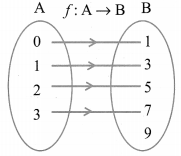(iv) A Graph f = {(x,f(x)/x ∈ A}
= {(0,1), (1,3), (2, 5), (3, 7)}Question 3.
State whether the graph represent a function. Use vertical line test.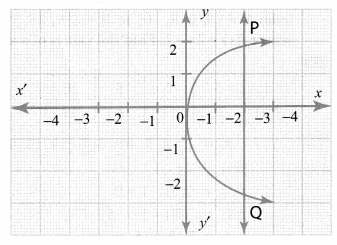Solution:
It is not a function as the vertical line PQ cuts the graph at two points.

Question 4.
Let f = {(2, 7), (3, 4), (7, 9), (-1, 6), (0, 2), (5,3)} be a function from A = {-1,0,2,3,5,7} to B = {2, 3, 4, 6, 7, 9}. Is this (i) an one-one function (ii) an onto function, (iii) both one- one and onto function?
Solution:
It is both one-one and onto function.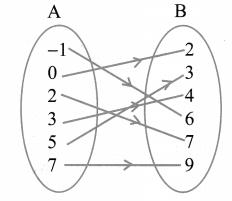All the elements in A have their separate images in B. All the elements in B have their preimage in A. Therefore it is one-one and onto function.

Question 5.
A function f: (-7,6) → R is defined as follows.Solution:(i) 2f(-4)+ 3f(2)
f(-4) = x + 5 = -4 + 5 = 1
2f(-4) = 2 × 1 = 2
f(2) = x + 5 = 2 + 5 = 7
3f(2) = 3(7) = 21
∴ 2f(-4) + 3f(2) = 2 + 21 = 23
f(-7) = x2 + 2x + 1
= (-7)2 + 2(-7) + 1
= 49 – 14 + 1 = 36
f(3) = x + 5 = -3 + 5 = 2
f(-7) – f(-3) = 36 – 2 = 34

Question 6.
f(x) = (1 + x)
f(x) = (2x – 1)
Show that fo(g(x)) = gof(x)
Solution:
f(x) = 1 + x
g(x) = (2x – 1)
fog(x) = f(g(x)) = f(2x – 1)
= 1 + 2x – 1 = 2x …(1)
gof(x) = g(f(x)) = g(1 + x) = 2(1 + x) = 1
= 2 + 2x – 1
= 2x + 1 …(2)
(1) ≠ (2)
∴ fog(x) + gof(x) It is verified.

Question 7.
Let A = {1, 2, 3, 4, 5}, B = N and f: A → B be defined by f(x) = x2. Find the range of f. Identify the type of function.
Solution:
A = {1,2,3,4,5}
B = {1,2,3,4….}
f: A → B, f(x) = x2
∴ f(1) = 12 = 1
f(2) = 22 = 4
f(3) = 32 = 9
f(4) = 42 = 16
f(5) = 52 = 25
∴ Range of f = {1,4,9,16,25)
Elements in A have been different elements in B. Therefore it is one-one function. But not all the elements in B have pre¬images in A. Therefore it is not on-to function.

Question 8.
A function f: [1, 6] → R is defined as follows: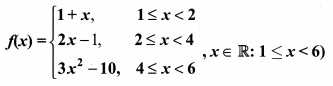Find the value of (i) f(5), (ii) f(3), (iii) f(2) – f(4).
Solution: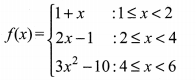Question 9.
The following table represents a function from A = {5, 6, 8, 10} to B = {19, 15, 9, 11}, where f(x) = 2x – 1. Find the values of a and b.
Solution: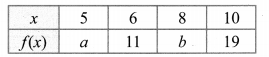A = {5,6,8, 10}, B = {19, 15,9, 11}
f(x) = 2x – 1
f(5) = 2(5) – 1 = 9
f(8) = 2(8) – 1 = 15
∴ a = 9, b = 15

Question 10.
If R = {(a, -2), (-5,6), (8, c), (d, -1)} represents the identity function, find the values of a,b,c and d.
Solution:
R = {(a,-2), (-5,b), (8,c), (d,-1)} represents the identity function.
a = -2, b = -5, c = 8, d = -1.